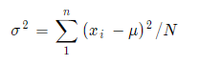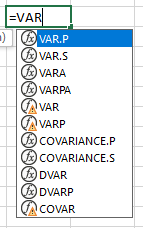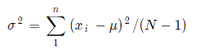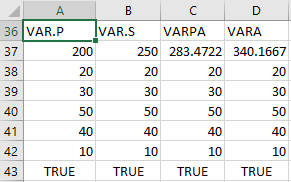# How To Calculate Variance in Excel?

• Last Updated : 09 May, 2021

First, let’s learn about Variance. In real-life scenarios, we have populations like marks of students in a particular subject, salaries of multiple employees in a company. Let us consider, in a company named ABC, we have 5 employees.

Now, it will tell us the expectation of standard deviation. In other words, the factor of difference in salaries.

The formula for std is –

\sigma^{2}  =   \sum_1^n (x_{i} -  \mu  )^{2}  / N

It will be shown asWhere x represents the value, and μ is the mean of the sample.

• Mean = 30
• N = 5
• Var = 200

Now, Let’s learn how to use it in Excel –

In Excel we have formulas for everything and as you might have guessed for var also.

We can have this list of formulas using ‘=’ symbol shown below1. VAR.P – It calculates the var assuming that the entire population is its argument. It returns an approximate value and is used with a large population. Uses the same formula shown above.

2. VAR.S – It calculates the var assuming that the sample of the population is its argument. It returns an approximate value and is used with a large population. Uses the same formula shown below

\sigma^{2}  =   \sum_1^n (x_{i} -  \mu  )^{2}  / (N -1)3. VARA – It includes text and logical symbols whereas the above 2 functions don’t include text and logical symbols. It is used for a sample of the population. Uses “n-1” formula.

4. VARPA – It includes text and logical symbols. It is used for the whole population. Uses “n” formula.

Let’s see the difference here,Here, the discrepancy occurs because VARPA and VARA read TRUE as 1 and VAR.P and VAR.S ignore it.

The formula goes like this –

1. =STDEV.P(A38:A43)        [Formula used in A37 cell]
2. =STDEV.S(B38:B43)        [Formula used in B37 cell]
3. =STDEVPA(C38:B43)        [Formula used in C37 cell]
4. =STDEVA(D38:B43)         [Formula used in D37 cell]

Note – VAR and VARP are for Excel 2007 or earlier. DVAR and DVARP are used for fetching data from Database.

My Personal Notes arrow_drop_up